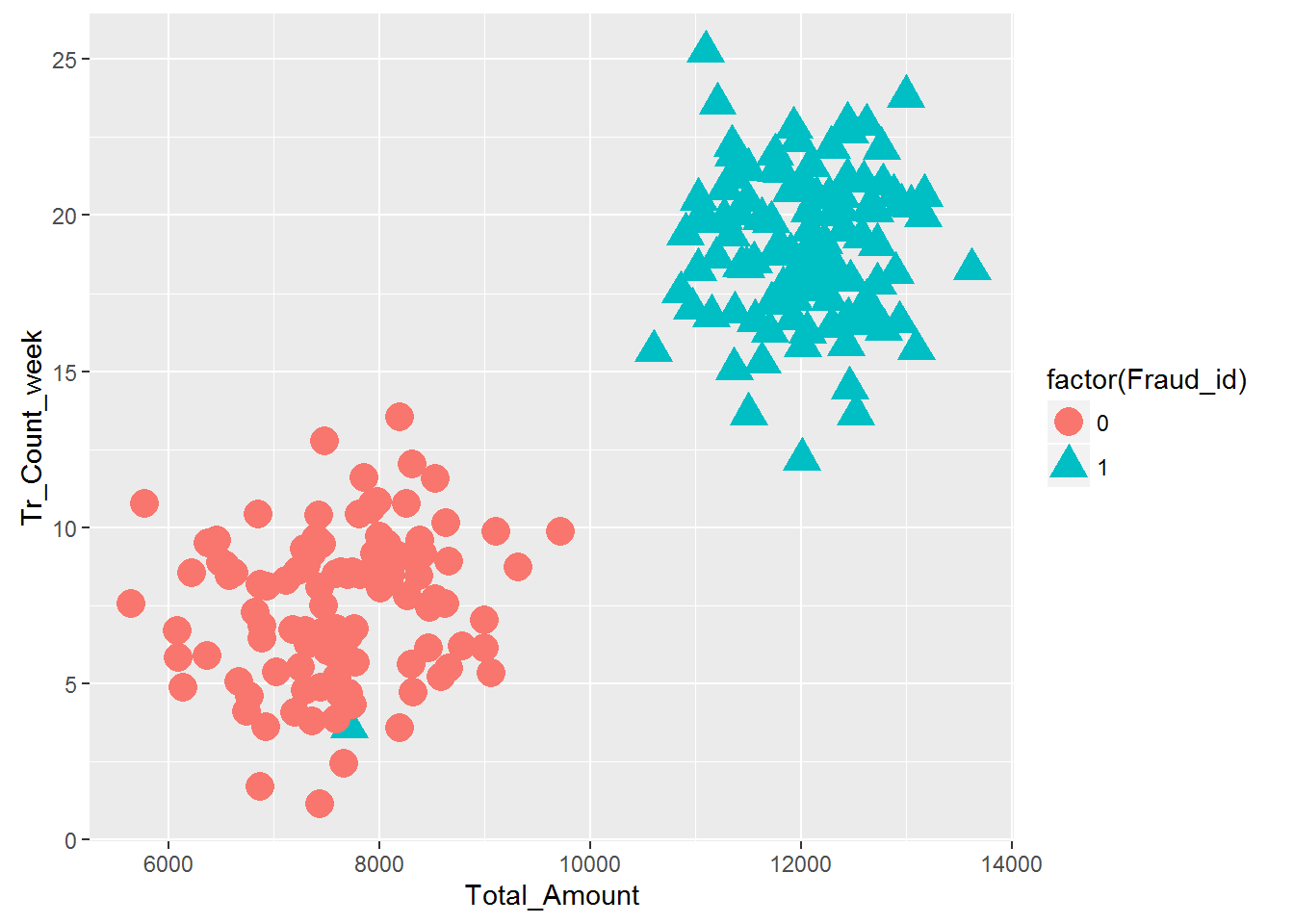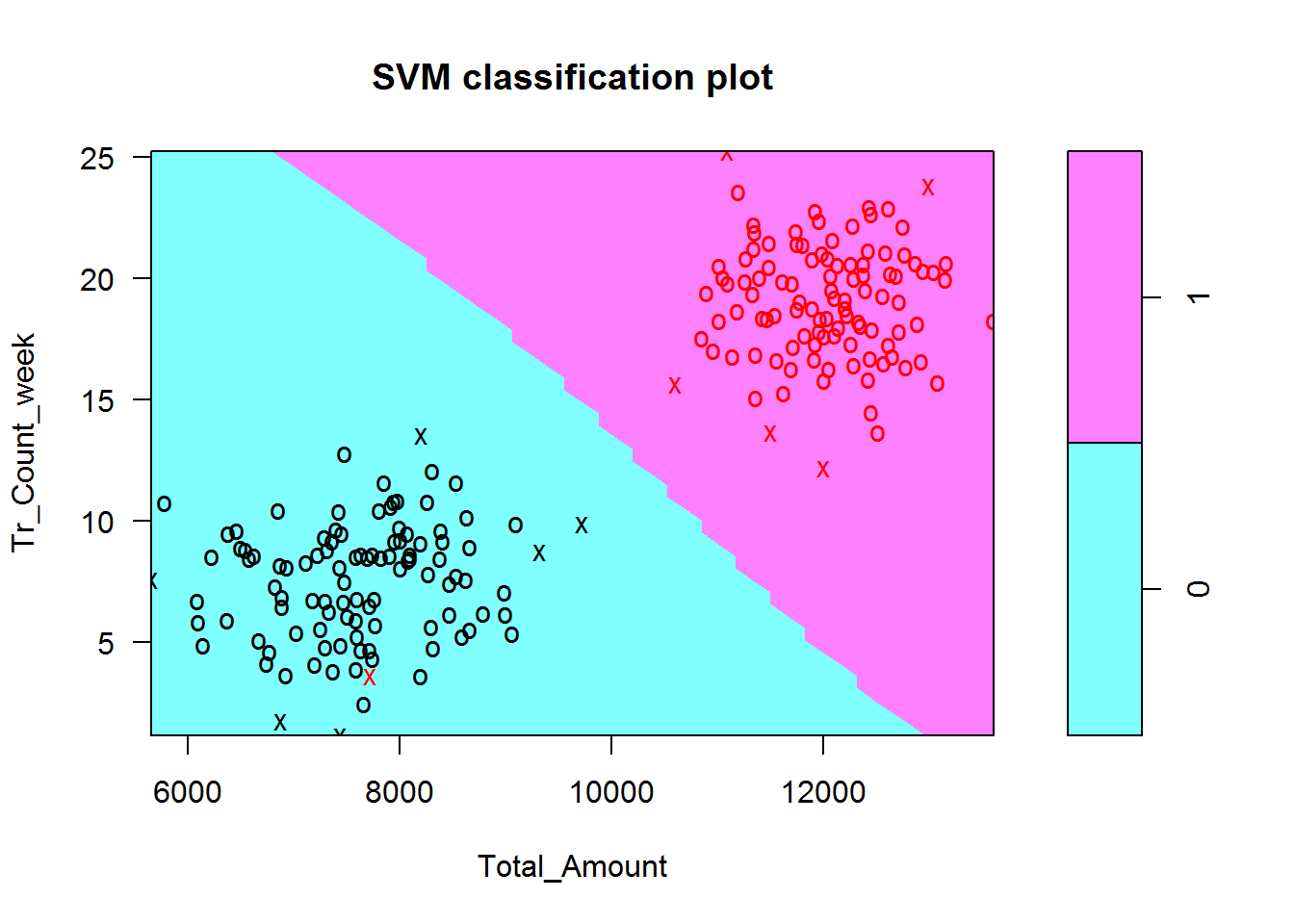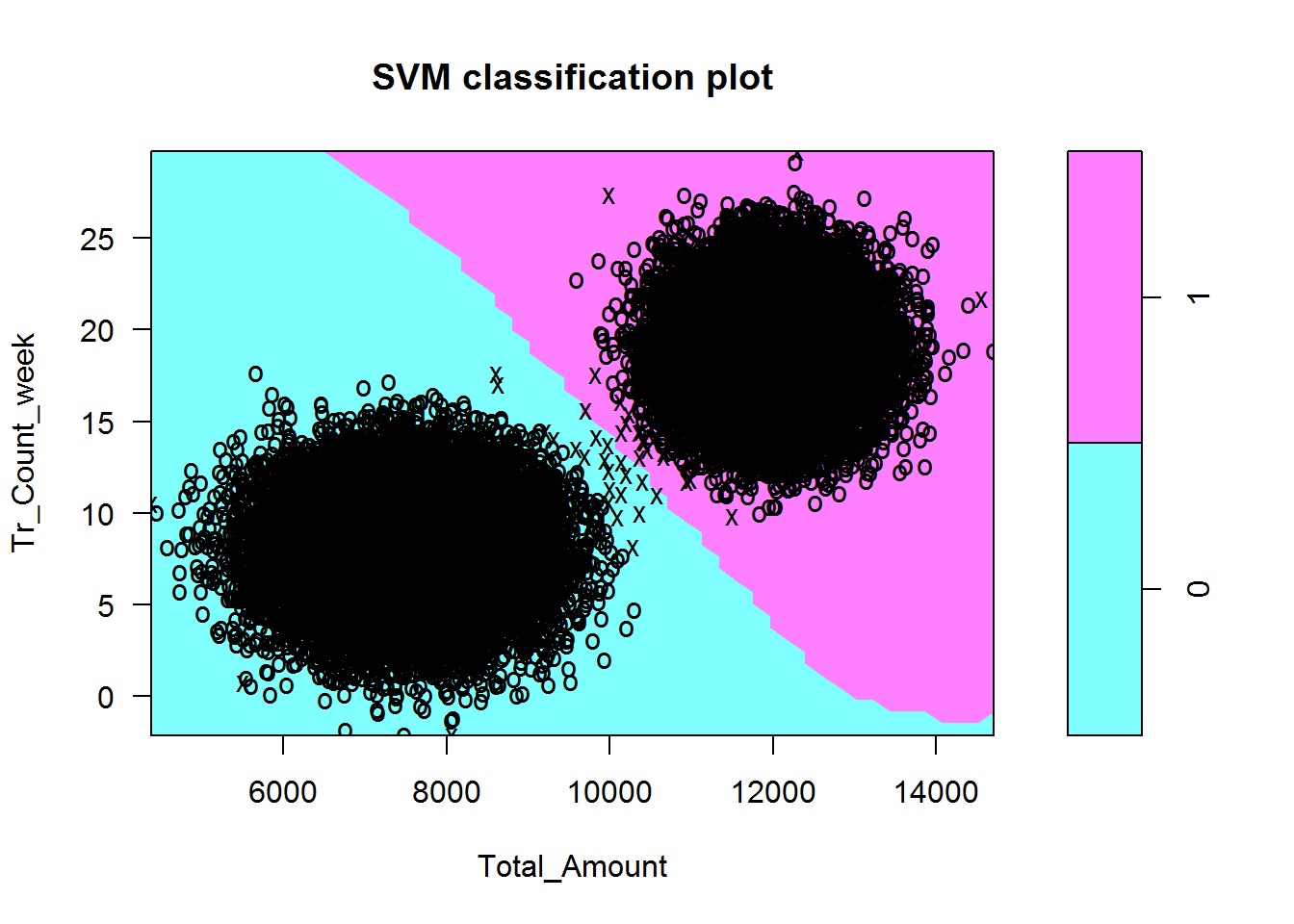• No products in the cart.

# 203.6.4 Building SVM model in R

##### Building a SVM model in R.

We discussed the SVM algorithm in our last post. In this post we will try to build a SVM classification model in R.

### LAB: First SVM Learning Problem

• Dataset: Fraud Transaction/Transactions_sample.csv
• Draw a classification graph that shows all the classes
• Build a SVM classifier
• Draw the classifier on the data plots
• Predict the (Fraud vs not-Fraud) class for the data points Total_Amount=11000, Tr_Count_week=15 & Total_Amount=2000, Tr_Count_week=4
• Draw a classification graph that shows all the classes
• Build a SVM classifier
• Draw the classifier on the data plots

### Solution

``````#SVM Building needs e1071 package
library(e1071)

#Converting the output into factor, otherwise SVM will fit a regression model
Transactions_sample\$Fraud_id<-factor(Transactions_sample\$Fraud_id)
``````##      id Total_Amount Tr_Count_week Fraud_id
## 1 16078      7294.60          4.79        0
## 2 41365      7659.53          2.45        0
## 3 11666      8259.29         10.77        0
## 4 11824     11630.25         15.29        1
## 5 36414     12286.63         22.18        1
## 6    90     12783.34         16.34        1``````
``````#SVM Model building
svm_model <- svm(Fraud_id~Total_Amount+Tr_Count_week, data=Transactions_sample)
summary(svm_model)``````
``````##
## Call:
## svm(formula = Fraud_id ~ Total_Amount + Tr_Count_week, data = Transactions_sample)
##
##
## Parameters:
##    SVM-Type:  C-classification
##        cost:  1
##       gamma:  0.5
##
## Number of Support Vectors:  12
##
##  ( 6 6 )
##
##
## Number of Classes:  2
##
## Levels:
##  0 1``````
``````#Plotting SVM Clasification graph o the data
ggplot(Transactions_sample)+geom_point(aes(x=Total_Amount,y=Tr_Count_week,color=factor(Fraud_id),shape=factor(Fraud_id)),size=5)````````````#Data With SVM model
plot(svm_model, Transactions_sample,Tr_Count_week~Total_Amount ) #x2~x1````````````#Prediction in SVM
new_data1<-data.frame(Total_Amount=11000, Tr_Count_week=15)
p1<-predict(svm_model, new_data1)
p1``````
``````## 1
## 1
## Levels: 0 1``````
``````new_data2<-data.frame(Total_Amount=2000, Tr_Count_week=4)
p2<-predict(svm_model, new_data2)
p2``````
``````## 1
## 0
## Levels: 0 1``````
``````#SVM on overall data
dim(Transactions)``````
``##  45000     4``
``````#Converting the output into factor, otherwise SVM will fit a regression model
svm_model_1 <- svm(Fraud_id~Total_Amount+Tr_Count_week, type="C", data=Transactions)
summary(svm_model_1)``````
``````##
## Call:
## svm(formula = Fraud_id ~ Total_Amount + Tr_Count_week, data = Transactions,
##     type = "C")
##
##
## Parameters:
##    SVM-Type:  C-classification
##        cost:  1
##       gamma:  0.5
##
## Number of Support Vectors:  44
##
##  ( 21 23 )
##
##
## Number of Classes:  2
##
## Levels:
##  0 1``````
``````#Plotting SVM Clasification graph
plot(svm_model_1, Transactions,Tr_Count_week~Total_Amount ) ``````The next post is about the Non-linear Decision Boundary.

21st June 2017

### 0 responses on "203.6.4 Building SVM model in R"

Statinfer Software Solutions LLP

Software Technology Parks of India,
NH16, Krishna Nagar, Benz Circle,